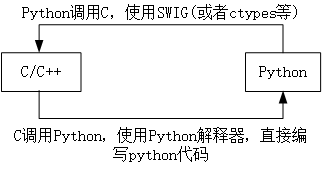### Android平台的Python-基础篇(一)-详细版

1. 前言

2. Python与C交互基础

2.1 C调用Python

2.1.1 简单使用

2.1.2 C调用Python函数

2.1.3 C调用Python基础API

2.2 Python 调用C

2.2.1 使用ctypes模块

2.2.2 使用C为Python编写拓展模块

# 1. 前言

(本文以Python3为例，Python3是未来，大家都懂的)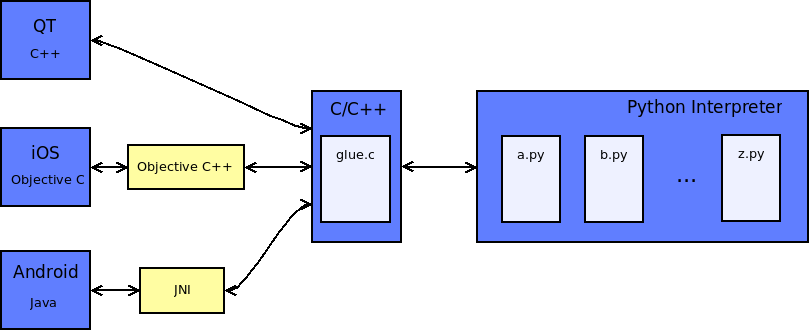# 2. Python与C交互基础

## 2.1 C调用Python

### 2.1.1 简单使用

（1）流程

• 初始化Python解析器。
• 执行Python代码，字符串，对象或模块。
• 关闭Python解析器。

（2）环境

• Visual Studio 2019；
• Anaconda version：4.8.3；
• Python version：anconda中的python 3.6.10。

（3）具体实现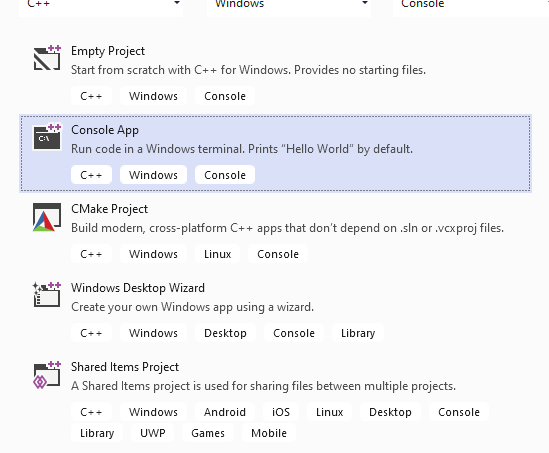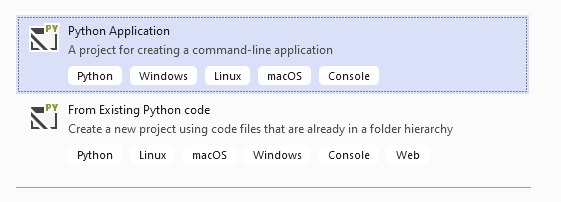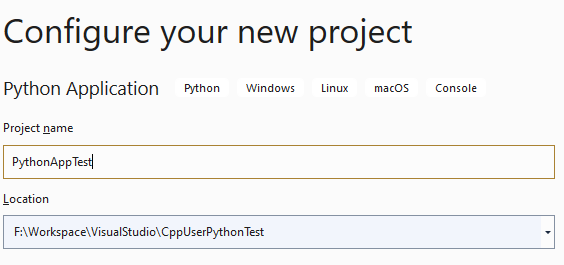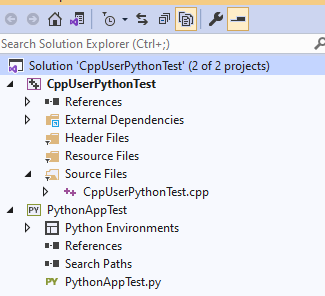``import datetime def printTime():    time_stamp = datetime.datetime.now()     print(time_stamp.strftime('%Y.%m.%d-%H:%M:%S'))    #print('invoke printTime:'+str(time.time()))    return (1,)#元组只有一个元素时，需在末尾加逗号``

`CppUserPythonTest.cpp`文件中添加调用Python的代码

``#include <Python.h> #include <iostream>#include <string> using namespace std; void InitPython(){    Py_Initialize(); /*初始化python解释器,告诉编译器要用的python编译器*/    if (!Py_IsInitialized())    {        printf("初始化失败！");        return;    }    PyRun_SimpleString("import sys"); //以下操作是路径设置，    PyRun_SimpleString("sys.argv = ['python.py']"); //添加    PyRun_SimpleString("sys.path.append('..')");    PyRun_SimpleString("sys.path.append('.')");    PyRun_SimpleString("sys.path.append('../PythonAppTest')"); //python文件的目录路径    PyRun_SimpleString("sys.path.append('./')");  }  int main(void){    InitPython();     PyRun_SimpleString("print('hello C !')");    PyRun_SimpleString("import PythonAppTest");    PyRun_SimpleString("PythonAppTest.printTime()");    Py_Finalize();//关闭Python解析器    return 0;}``

``PyRun_SimpleString(“execfile(“test.py”)”)``

（4）项目配置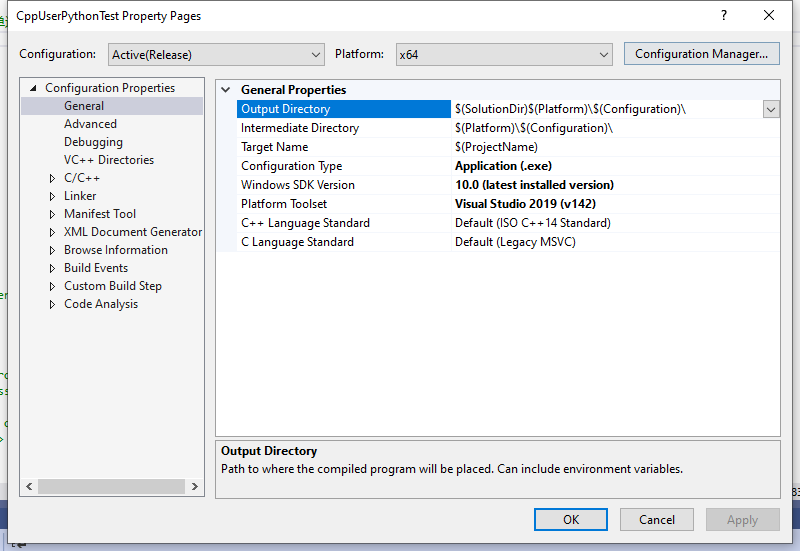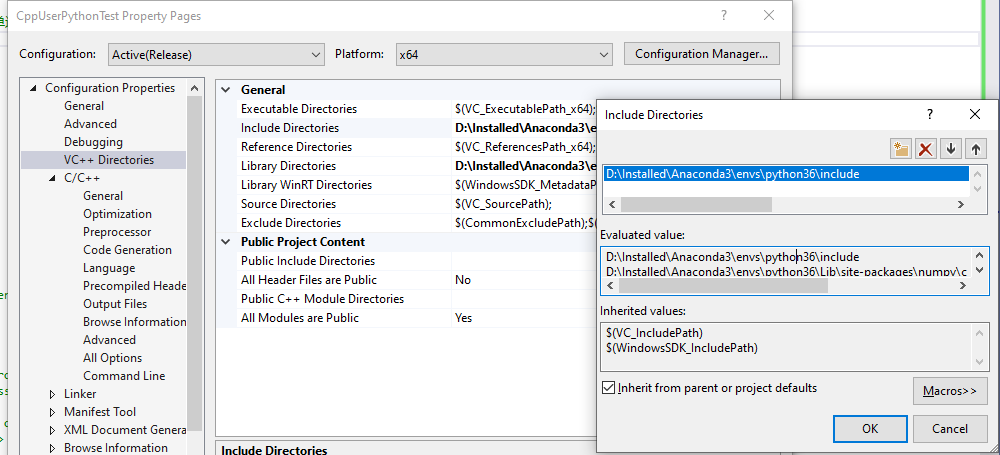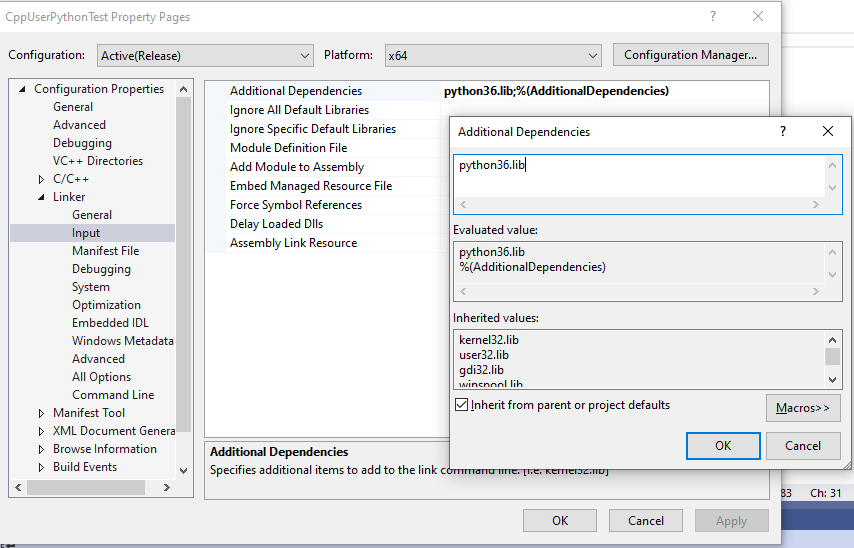（5）执行程序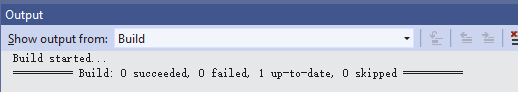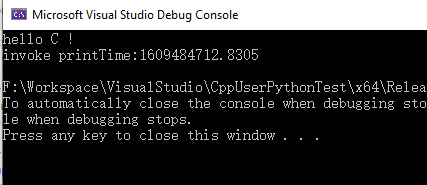### 2.1.2 C调用Python函数

`` #include <Python.h>#include <iostream>#include <string> using namespace std; void InitPython(){    Py_Initialize(); /*初始化python解释器,告诉编译器要用的python编译器*/    if (!Py_IsInitialized())    {        printf("初始化失败！");        return;    }    PyRun_SimpleString("import sys"); //以下操作是路径设置，    PyRun_SimpleString("sys.argv = ['python.py']"); //添加    PyRun_SimpleString("sys.path.append('..')");    PyRun_SimpleString("sys.path.append('.')");    PyRun_SimpleString("sys.path.append('../PythonAppTest')"); //python文件的目录路径    PyRun_SimpleString("sys.path.append('./')");} int main(void){	PyObject* module_name, * module, * func, * dic;	const char* fun_name = "printTime";//需调用的Python函数名	PyObject* resultValue; 	InitPython();	//导入Python 模块并检验	module_name = Py_BuildValue("s", "PythonAppTest");	module = PyImport_Import(module_name); 	if (!module)	{		printf("import test failed!");		return -1;	} 	//获取模块中的函数列表，是一个函数名和函数地址对应的字典结构	dic = PyModule_GetDict(module);	if (!dic)	{		printf("failed !\n");		return -1;	} 	func = PyDict_GetItemString(dic, fun_name);	if (!PyCallable_Check(func))	{		printf("not find %s\n", fun_name);		return -1;	} 	int r;	//获取Python函数返回值，是一个元组对象	resultValue = PyObject_CallObject(func, NULL);	PyArg_ParseTuple(resultValue, "i", &r);	printf("result :%d\n", r); 	Py_DECREF(module);	Py_DECREF(dic);	Py_Finalize();	return 0;}``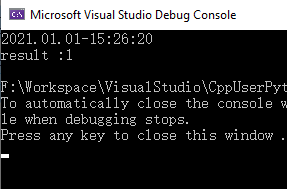### 2.1.3 C调用Python基础API

C 调用Python API Python 对应
`PyImport_ImportModel` import module
`PyImport_ReloadModule` reload(module)
`PyImport_GetModuleDict` module._dict_
`PyDict_GetItemString` dict[key]
`PyDict_SetItemString` dict[key] = value
`PyDict_New` dict = {}
`PyObject_GetAttrString` getattr(obj, attr)
`PyObject_SetAttrString` setattr(obj, attr, val)
`PyObject_CallObject` funcobj(*argstuple)
`PyEval_CallObject` funcobj(*argstuple)
`PyRun_String` eval(exprstr) , exec(stmtstr)
`PyRun_File` exec(open(filename().read())

（1）Py_BuildValue()函数

format及转换格式，类似与C语言中%d,%f，后面的不定参数对应前面的格式，具体格式如下：

“s”(string) [char *] ：将C字符串转换成Python对象，如果C字符串为空，返回NONE。

“s#”(string) [char *, int] :将C字符串和它的长度转换成Python对象，如果C字符串为空指针，长度忽略，返回NONE。

“z”(string or None) [char *] :作用同”s”。

“z#” (stringor None) [char *, int] :作用同”s#”。

“i”(integer) [int] :将一个C类型的int转换成Python int对象。

“b”(integer) [char] :作用同”i”。

“h”(integer) [short int] ：作用同”i”。

“l”(integer) [long int] :将C类型的long转换成Pyhon中的int对象。

“c”(string of length 1) [char] ：将C类型的char转换成长度为1的Python字符串对象。

“d”(float) [double] :将C类型的double转换成python中的浮点型对象。

“f”(float) [float] :作用同”d”。

“O&”(object) [converter, anything] ：将任何数据类型通过转换函数转换成Python对象，这些数据作为转换函数的参数被调用并且返回一个新的Python对象，如果发生错误返回NULL。

“(items)”(tuple) [matching-items] ：将一系列的C值转换成Python元组。

“[items]”(list) [matching-items] ：将一系列的C值转换成Python列表。

“{items}”(dictionary) [matching-items] ：将一系类的C值转换成Python的字典，每一对连续的C值将转换成一个键值对。

``      Py_BuildValue("") None      Py_BuildValue("i",123) 123      Py_BuildValue("iii",123, 456, 789) (123, 456, 789)      Py_BuildValue("s","hello") 'hello'      Py_BuildValue("ss","hello", "world") ('hello', 'world')      Py_BuildValue("s#","hello", 4) 'hell'      Py_BuildValue("()") ()      Py_BuildValue("(i)",123) (123,)           Py_BuildValue("(ii)",123, 456) (123, 456)      Py_BuildValue("(i,i)",123, 456) (123, 456)      Py_BuildValue("[i,i]",123, 456) [123, 456]            Py_BuildValue("{s:i,s:i}", "abc",123, "def", 456) {'abc': 123, 'def': 456}      Py_BuildValue("((ii)(ii))(ii)", 1, 2, 3, 4, 5, 6) (((1, 2), (3, 4)), (5, 6))``

（2）PyArg_ParseTuple函数

``  args:一般为Python程序返回的元组。  format:与Py_BulidValue类型，就不在累述咯。     元组操作函数：  因为程序之间传递的参数，大多数为Tuple类型，所以有专门的函数来操作元组：     PyAPI_FUNC(PyObject *) PyTuple_New(Py_ssize_t size);  解释：新建一个参数列表（调试了下，发现其实是用链表实现的），size列表为长度的宽度     PyAPI_FUNC(Py_ssize_t) PyTuple_Size(PyObject *);  解释：获取该列表的大小     PyAPI_FUNC(PyObject *) PyTuple_GetItem(PyObject *, Py_ssize_t);  解释：获取该列表某位置的值     PyAPI_FUNC(int) PyTuple_SetItem(PyObject *,Py_ssize_t, PyObject *);  解释：设置该列表此位置的值。如PyTuple_SetItem(pyParams,1,Py_BuildValue("i",2));设置第2个位置的值为2的整数。	``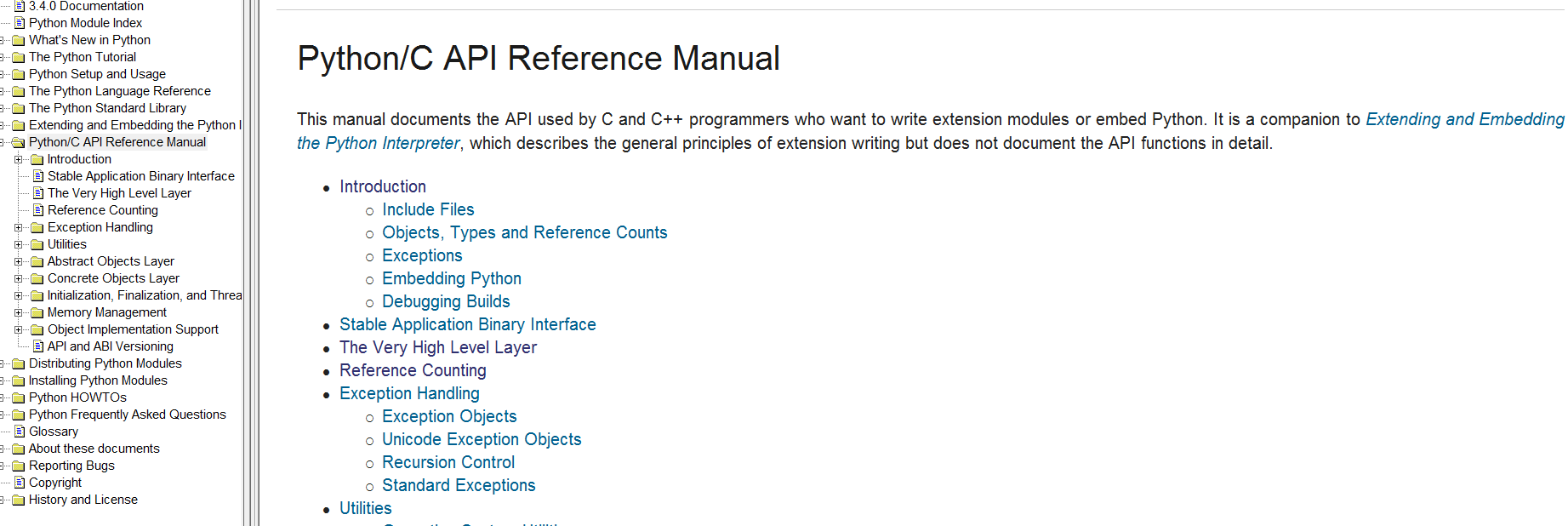## 2.2 Python 调用C

Python调用C的方法有多种，见https://blog.csdn.net/jwh_bupt/article/details/8287416。这里只介绍种方式：

### 2.2.1 使用ctypes模块

Python文档有详细示例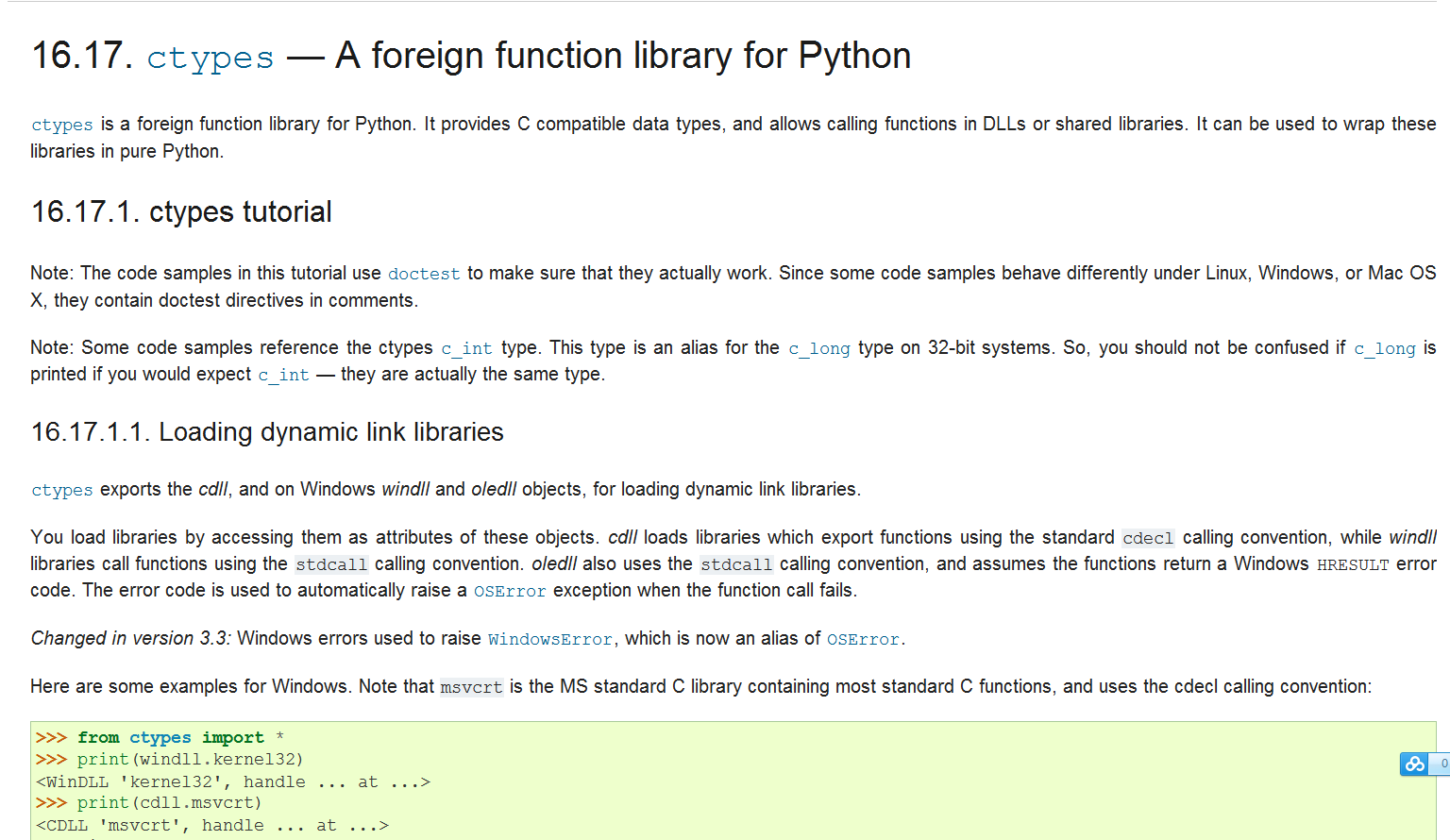### 2.2.2 使用C为Python编写拓展模块

Python之所以如此强大，正是由于可以使用C\C++为其编写拓展模块，手动编写拓展模块的方式稍微有些繁琐，可借用SWIG自动实现，简洁快速。更多详细的SWIG用法，见其官方文档。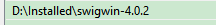（1）直接使用SWIG和命令行

（1.1）创建源文件和swig接口文件。

``//mytest.hint add(int a,int b);int sub(int a,int b);``

``//mytest.cppint add(int a, int b){   return a+ b;}   int sub(int a,int b){ return a - b;}``

``%module mytest%{#define SWIG_WITH_INIT#include "mytest.h"%}%include "mytest.h"``

``%inline %{包含导出的函数%}``

%module 后面的名字是被封装的模块名称。封装口，python通过这个名称加载程序。

%{  %}之间所添加的内容，一般包含此文件需要的一些函数声明和头文件。

（1.2） 在命令行中运行

``swig -c++ -python mytest.i``

``swig -python mytest.i``

（1.3）编写setup.py文件

``from distutils.core import setupfrom distutils.extension import Extension test_module = Extension('_mytest', sources=['mytest_wrap.cxx', 'mytest.cpp'],) setup(name = 'mytest',    version = '0.1',    author = 'SWIG Docs',    description = 'Simple swig pht from docs',    ext_modules = [test_module],    py_modules = ['mytest'])``

``python setup.py build``

（1.4）编写python脚本调用C++

``import mytest a = mytest.add(1, 2)print(a) b = mytest.sub(2, 1)print(b)``

## 2.3 C与Python交互总结